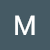# [Leet Code] Check if All Characters Have Equal Number of Occurrences

Problem:

Given a string `s`, return `true` if `s` is a good string, or `false` otherwise.

A string `s` is good if all the characters that appear in `s` have the same number of occurrences (i.e., the same frequency).

Example 1:

`Input: s = "abacbc"Output: trueExplanation: The characters that appear in s are 'a', 'b', and 'c'. All characters occur 2 times in s.`

Example 2:

`Input: s = "aaabb"Output: falseExplanation: The characters that appear in s are 'a' and 'b'. …`

# [LeetCode] Replace All Digits with Characters

Problem:

You are given a 0-indexed string `s` that has lowercase English letters in its even indices and digits in its odd indices.

There is a function `shift(c, x)`, where `c` is a character and `x` is a digit, that returns the `xth` character after `c`.

• For example, `shift('a', 5) = 'f'` and `shift('x', 0) = 'x'`.

For every odd index `i`, you want to replace the digit `s[i]` with `shift(s[i-1], s[i])`.

Return `s` after replacing all digits. It is guaranteed that `shift(s[i-1], s[i])` will never exceed `'z'`.

Example 1:

`Input: s = "a1c1e1"Output: "abcdef"Explanation: The digits are…`

# [LeetCode] Check if Word Equals Summation of Two Words

Problem:

The letter value of a letter is its position in the alphabet starting from 0 (i.e. `'a' -> 0`, `'b' -> 1`, `'c' -> 2`, etc.).

The numerical value of some string of lowercase English letters `s` is the concatenation of the letter values of each letter in `s`, which is then converted into an integer.

• For example, if `s = "acb"`, we concatenate each letter's letter value, resulting in `"021"`. After converting it, we get `21`.

You are given three strings `firstWord`, `secondWord`, and `targetWord`, each consisting of lowercase English letters `'a'` through `'j'` inclusive.

Return…

# [LeetCode] Truncate Sentence

Problem:

A sentence is a list of words that are separated by a single space with no leading or trailing spaces. Each of the words consists of only uppercase and lowercase English letters (no punctuation).

• For example, `"Hello World"`, `"HELLO"`, and `"hello world hello world"` are all sentences.

You are given a sentence `s`​​​​​​ and an integer `k`​​​​​​. You want to truncate `s`​​​​​​ such that it contains only the first `k`​​​​​​ words. Return `s`​​​​​​ after truncating it.

Example 1:

`Input: s = "Hello how are you Contestant", k = 4Output: "Hello how are you"Explanation:The words in s are…`

# [LeetCode] Sorting the Sentence

Problem:

A sentence is a list of words that are separated by a single space with no leading or trailing spaces. Each word consists of lowercase and uppercase English letters.

A sentence can be shuffled by appending the 1-indexed word position to each word then rearranging the words in the sentence.

• For example, the sentence `"This is a sentence"` can be shuffled as `"sentence4 a3 is2 This1"` or `"is2 sentence4 This1 a3"`.

Given a shuffled sentence `s` containing no more than `9` words, reconstruct and return the original sentence.

Example 1:

`Input: s = "is2 sentence4 This1 a3"Output…`

# [Leet Code] Roman to Integer

Problem:

Roman numerals are represented by seven different symbols: `I`, `V`, `X`, `L`, `C`, `D` and `M`.

`Symbol       ValueI             1V             5X             10L             50C             100D             500M             1000`

For example, `2` is written as `II` in Roman numeral, just two one's added together. `12` is written as `XII`, which is simply `X + II`. The number `27` is written as `XXVII`, which is `XX + V + II`.

Roman numerals are usually written largest to smallest from left to right. However, the numeral for four is not `IIII`. Instead, the number four is…

# [Leet Code] Design Authentication Manager

Problem:

There is an authentication system that works with authentication tokens. For each session, the user will receive a new authentication token that will expire `timeToLive` seconds after the `currentTime`. If the token is renewed, the expiry time will be extended to expire `timeToLive` seconds after the (potentially different) `currentTime`.

Implement the `AuthenticationManager` class:

• `AuthenticationManager(int timeToLive)` constructs the `AuthenticationManager` and sets the `timeToLive`.
• `generate(string tokenId, int currentTime)` generates a new token with the given `tokenId` at the given `currentTime` in seconds.
• `renew(string tokenId, int currentTime)` renews the unexpired token with the given `tokenId` at the given `currentTime` in…# [Leet Code] The K Weakest Rows in a Matrix

Problem:

You are given an `m x n` binary matrix `mat` of `1`'s (representing soldiers) and `0`'s (representing civilians). The soldiers are positioned in front of the civilians. That is, all the `1`'s will appear to the left of all the `0`'s in each row.

A row `i` is weaker than a row `j` if one of the following is true:

• The number of soldiers in row `i` is less than the number of soldiers in row `j`.
• Both rows have the same number of soldiers and `i < j`.

Return the indices of the `k` weakest rows…

# [Leet Code] Merge Strings Alternately

Problem:

You are given two strings `word1` and `word2`. Merge the strings by adding letters in alternating order, starting with `word1`. If a string is longer than the other, append the additional letters onto the end of the merged string.

Return the merged string.

Example 1:

`Input: word1 = "abc", word2 = "pqr"Output: "apbqcr"Explanation: The merged string will be merged as so:word1:  a   b   cword2:    p   q   rmerged: a p b q c r`

Example 2:

`Input: word1 = "ab", word2 = "pqrs"Output: "apbqrs"Explanation: Notice that as word2 is longer, "rs" is appended…`## Matthew Boyd

Learning, and posting my findings!